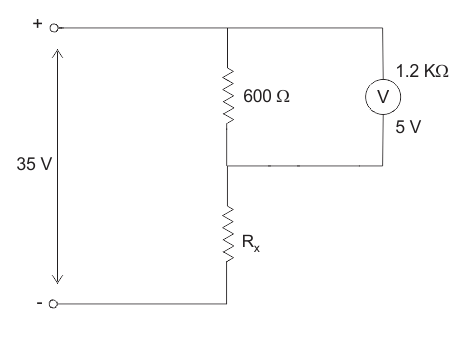# MCQs on Electrical And Electronics Measurements

##### Page 13 of 19. Go to page 1 2 3 4 5 6 7 8 9 10 11 12 13 14 15 16 17 18 19
01․ Assertion(A): A low power factor wattmeter has special constructional features to ensure accurate measurment. Reason(R): Extension of wattmeter range in DC circuit can be easily done by using current and potential transformer
A is false R is true.
A is true R is false.
Both A and R is true and R is correct explanation of A C.
Both A and R is true and R is not correct explanation of A.

Current transformer and potential transformer cannot be used in DC.

02․ In which of the transformer is the secondary nearly short circuited under normal operating condition?
PT.
Power transformer.
CT.
Distribution transformer.

CT is operated with secondary short circuited, otherwise a high emf would be induced across secondary.

03․ Figure show an RC potentiometer to measure AC voltage. It is desire that Vo/Vi should be independent of frequency. The value of C shoud be10 µF.
11 µF.
0.1 µF.
0.09 µF.

Since resistance are in the ratio of 10:1. Capacitance should be in the ratio of 1:10.

04․ To increase Q factor of a coil, the wire should be
thick.
long.
thin.
long and thin.

Q is high if resistance is low. Therefore, wire should be thick.

05․ The use of thermocouple meters for AC measurement leads to scale which is
linear.
square law.
expontial.
logarithmic.

Thermocouple meter is based on heating effect which varies as the square of current flowing.

06․ Which of following is not correct?
Voltmeter should have very high resistance.
An electronic voltmeter draw appreciable current from the source.
An ammeter should have a very low resitance.
A shunt should have a very low resistance.

An electronic voltmeter draws negligible current from the source.

07․ The coil of a moving iron instrument has a resistance of 500 ohm and inductance of 1 H. It reads 250 V when a 250 V DC is applied. It series resistance is 2000 ohms its reading when fed by 250 V, 50 Hz AC will be
260 V.
252 V.
250 V.
248 V.

Meter current for DC is Meter current for DC is IDC = 250/(2000 + 500) = 0.1A. Meter current for AC is IAC = 250/(2500 + j314.16) = 0.0992A. Therefore Reading = (0.0992/0.1) × 250 = 248 V.

08․ In a CRO which of following is not a part of electron gun?
Grid
Cathode
X-Y plates
Accelerating anode

In CRO, electron gun consist of cathode, grid and accelerating anode.

09․ Which of following voltmeters would you use for measuring voltage across 20KΩ resistance
Voltmeter having sensitivity of 10 KW/V.
Voltmeter having a resistance of 5 ΩK.
Voltmeter having sensitivity of 1 KW/V.
None of above.

The resistance of voltmeter should be very high as compared to 20 KΩ. As it results the sensitivity of voltmeter will be high.

10․ A PMMC voltmeter is connected across a series combination of a DC voltage source V1 = 2 V and an AC voltage source V2 = 3sin4t, the voltmeters reads
2 V.
5 V.
(2 + √3/2)V.
0 V.

The permanent magnet moving coil measures the DC value only. The torque will be reversed if the connected to AC, the deflection responds to the mean torque which is zero.

<<<1112131415>>>